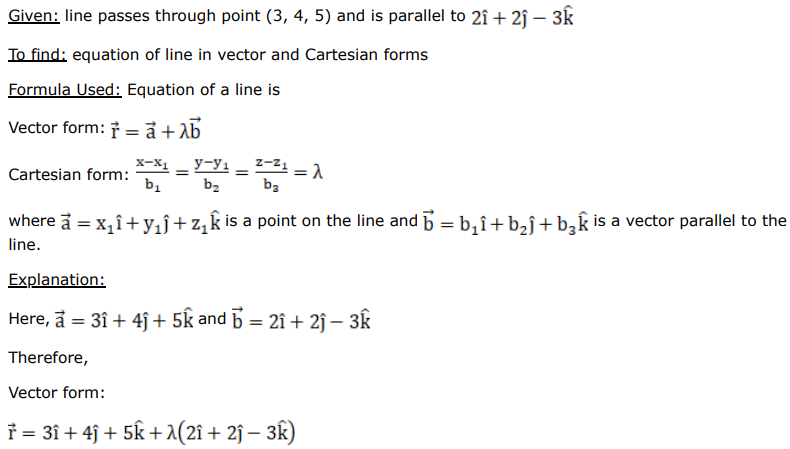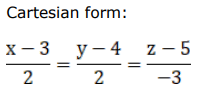# A line passes through the pointQuestion:

A line passes through the point $(3,4,5)$ and is parallel to the vector $(2 \hat{\mathrm{i}}+2 \hat{\mathrm{j}}-3 \hat{\mathrm{k}})$. Find the equations of the line in the vector as well as Cartesian forms.

Solution: